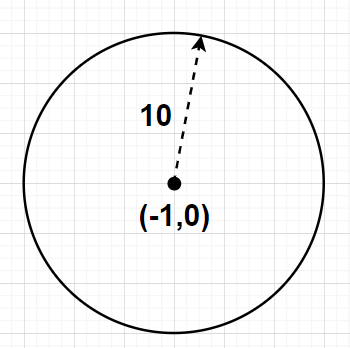QUESTION

# If $\left| z \right| = 2$, then the points representing the complex numbers $- 1 + 5z$ will lie on${\text{A}}{\text{.}}$ Circle${\text{B}}{\text{.}}$ Straight line${\text{C}}{\text{.}}$ Parabola${\text{D}}{\text{.}}$ None of theseHint- Here, we will proceed by letting any complex number $z' = x + iy = - 1 + 5z$ and then taking the modulus on both the sides and then solving using the formulas $\left| {az} \right| = a\left| z \right|$ and $\left| z \right| = \sqrt {{u^2} + {w^2}}$ for any complex number $z = u + iw$.

Given, Modulus of complex number z is $\left| z \right| = 2{\text{ }} \to {\text{(1)}}$
Let $z' = x + iy$ be any complex number which lies on the locus represented by the complex numbers $- 1 + 5z$

Given, $z' = - 1 + 5z \\ \Rightarrow z' + 1 = 5z \\$

By taking the modulus of the complex numbers on both the sides, we get

$\Rightarrow \left| {z' + 1} \right| = \left| {5z} \right|$

Using the formula $\left| {az} \right| = a\left| z \right|$ where a is any real number and z is

any complex number in the above equation, we get

$\Rightarrow \left| {z' + 1} \right| = 5\left| z \right|$

Using equation (1) in the above equation, we get

$\Rightarrow \left| {z' + 1} \right| = 5 \times 2 \\ \Rightarrow \left| {z' + 1} \right| = 10 \\$

By substituting $z' = x + iy$ in the above equation, we get

$\Rightarrow \left| {x + iy + 1} \right| = 10 \\ \Rightarrow \left| {\left( {x + 1} \right) + iy} \right| = 10{\text{ }} \to {\text{(2)}} \\$

For any complex number $z = u + iw$, the modulus of this complex number is given by

$\left| z \right| = \sqrt {{u^2} + {w^2}} {\text{ }} \to {\text{(3)}}$

Using the formula given by equation (3) in equation (2), we get

$\Rightarrow \sqrt {{{\left( {x + 1} \right)}^2} + {y^2}} = 10$

Squaring both sides of the above equation, we get

$\Rightarrow {\left( {\sqrt {{{\left( {x + 1} \right)}^2} + {y^2}} } \right)^2} = {\left( {10} \right)^2} \\ \Rightarrow {\left( {x + 1} \right)^2} + {y^2} = 100{\text{ }} \to {\text{(4)}} \\$

As we know that the general equation of a circle having $\left( {{x_1},{y_1}} \right)$ and

having radius r is given by

${\left( {x - {x_1}} \right)^2} + {\left( {y - {y_1}} \right)^2} = {r^2}{\text{ }} \to {\text{(5)}}$

Representing the equation (4) in the same form as given by equation (5), we have

$\Rightarrow {\left( {x - \left( { - 1} \right)} \right)^2} + {\left( {y - 0} \right)^2} = {\left( {10} \right)^2}{\text{ }} \to {\text{(6)}}$

The above equation (6) is the equation of a circle with the centre at $\left( { - 1,0} \right)$

and having a radius of 10.

Therefore, the points representing the complex numbers $- 1 + 5z$ will lie on a circle having

a centre at $\left( { - 1,0} \right)$ and having a radius of 10.

Hence, option A is correct

Note- Any complex number $z = u + iw$ contains u as the real part of the complex number z and w as the imaginary part of the complex number. Also, all the real numbers are complex numbers because any real number b can be written as $b = b + i\left( 0 \right)$ where the real part of the corresponding complex number is b itself and the corresponding imaginary part is zero.